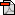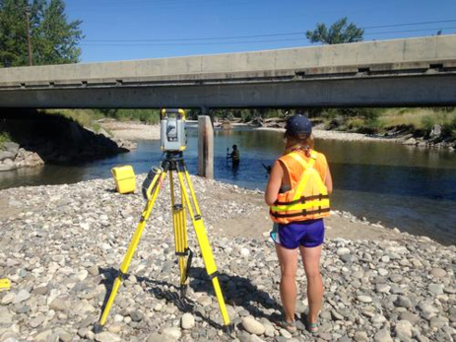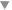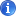GETSI Teaching Materials >Flood Hazards > Unit 3: Channel Capacity and Manning's Equation
GETSI's Earth-focused Modules for Undergraduate Classroom and Field CoursesLearn More
This module is part of a growing collection of classroom-tested materials developed by GETSI. The materials engage students in understanding the earth system as it intertwines with key societal issues. The collection is freely available and ready to be adapted by undergraduate educators across a range of courses including: general education or majors courses in Earth-focused disciplines such as geoscience or environmental science, social science, engineering, and other sciences, as well as courses for interdisciplinary programs.
Explore the Collection »Download

# Unit 3: Channel Capacity and Manning's Equation

## Summary

A flood occurs when the flow rate in a river exceeds the capacity of a channel to transmit water downstream within its banks. How much water can a channel transmit? Answering this question requires measurements of channel and floodplain topography, coupled with the application of the physics of flow in channels. These complex concepts are embodied in the well-known Manning's Equation. In this unit, students evaluate the geometry of river channels and floodplains using LIDAR-derived data and compute the depths and velocities of flow rates within channels using Manning's equation.

Used this activity? Share your experiences and modifications

## Learning Goals

### Unit 3 Learning Outcomes×Show captionHideTraditional river surveyors taking measurements of channel and floodplain topography. A variety of surveying methods such as the one shown here are highlighted in this unit. From Steve Holnbeck, Wyoming-Montana Water Science Center.Students will be able to:

• Describe geodetic methods used to measure channel and floodplain geometry
• Describe the basic physical concepts underlying Manning's equation
• Apply Manning's equation to compute the flow rate that a channel of known geometry can transmit

## Context for Use

This unit provides basic information about floodplain geometry and Manning's equation. The PowerPoint files provide just enough information to connect Unit 2 on flood frequency analysis with Unit 4: Hydraulic Modeling and Flood Inundation Mapping using HEC-RAS on hydraulic modeling of floodplains. In Unit 4, Manning's equation is embedded inside a rather complex hydraulic model. This unit gives students a chance to compute Manning's equation using real data so that Unit 4 is more understandable. The student exercise could also be used in other classes that go into deeper detail about channel hydraulics and floods such as geoscience courses on geomorphology or engineering courses on open channel flow.

## Description and Teaching Materials

This unit includes two PowerPoint presentations that could be used together in a single class period. The first gives a basic introduction to geodetic methods used in floodplain analysis so that students have an understanding of how the data were collected. The second gives a brief introduction to Manning's equation. Both could be easily expanded by an instructor to tailor the level of detail to a specific course. The student exercise presents a very prescriptive example of how to use Manning's equation with real survey data, and then asks students to repeat the exercise on the other cross-section. Final answers are provided for the first cross-section so that students can check their work and make sure they develop the correct formulae as they work through calculating wetted perimeter and cross-sectional area.

## Teaching Notes and Tips

• If students have not used Excel before, they could struggle with some of the computations. Instructors should ensure that the higher learning objectives about understanding Manning's equation and floods are not lost while making the calculations in Excel. Certain Excel functions such as use of \$, abs, sum, etc. may need to be reviewed if students are not highly familiar with spreadsheets.

## Assessment

A simple formative assessment is presented in the Manning PowerPoint. Students should complete a Manning's calculation with simple geometry before working through the Excel example. This formative assessment should not be graded, but instructors should ensure that each student understands each variable in Manning's equation. The student exercise forms the summative assessment for this unit. Instructors should just check for completion of the Boise River example, but grade the Wabash project for quality and comprehension.

## References and Resources

Already used some of these materials in a course?
Let us know and join the discussion »

Considering using these materials with your students?